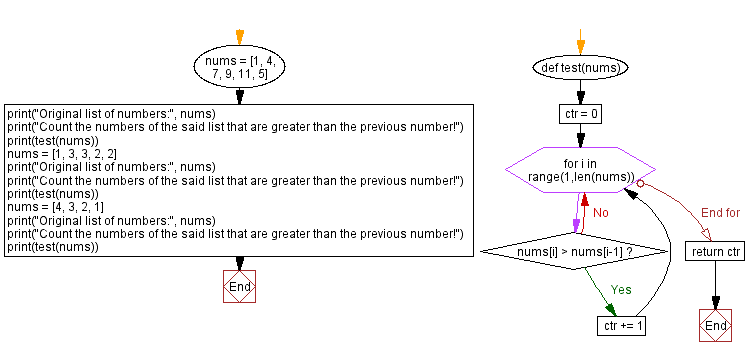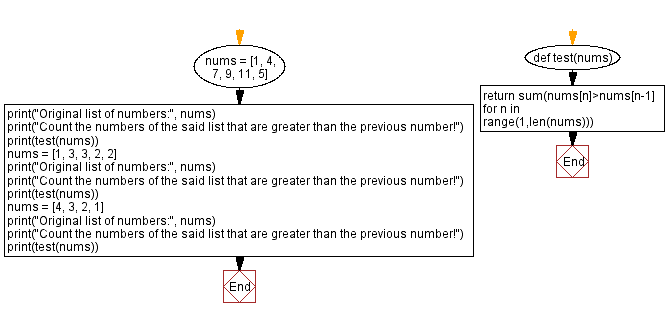﻿ Python - Check the numbers that are higher than the previous

# Python Exercise: Check the numbers that are higher than the previous

## Python Basic - 1: Exercise-148 with Solution

A Python list contains some positive integers. Write a Python program to count the numbers that are greater than the previous number on the list.

Sample Data:

([1, 4, 7, 9, 11, 5]) -> 4
([1, 3, 3, 2, 2]) -> 1
([4, 3, 2, 1]) -> 0

Sample Solution-1:

Python Code:

``````def test(nums):
ctr = 0
for i in range(1,len(nums)):
if nums[i] > nums[i-1]:
ctr += 1
return ctr

nums = [1, 4, 7, 9, 11, 5]
print("Original list of numbers:", nums)
print("Count the numbers of the said list that are greater than the previous number!")
print(test(nums))
nums = [1, 3, 3, 2, 2]
print("Original list of numbers:", nums)
print("Count the numbers of the said list that are greater than the previous number!")
print(test(nums))
nums = [4, 3, 2, 1]
print("Original list of numbers:", nums)
print("Count the numbers of the said list that are greater than the previous number!")
print(test(nums))
``````

Sample Output:

```Original list of numbers: [1, 4, 7, 9, 11, 5]
Count the numbers of the said list that are greater than the previous number!
4
Original list of numbers: [1, 3, 3, 2, 2]
Count the numbers of the said list that are greater than the previous number!
1
Original list of numbers: [4, 3, 2, 1]
Count the numbers of the said list that are greater than the previous number!
0
```

Flowchart:Sample Solution-2:

Python Code:

``````def test(nums):
return sum(nums[n]>nums[n-1] for n in range(1,len(nums)))
nums = [1, 4, 7, 9, 11, 5]
print("Original list of numbers:", nums)
print("Count the numbers of the said list that are greater than the previous number!")
print(test(nums))
nums = [1, 3, 3, 2, 2]
print("Original list of numbers:", nums)
print("Count the numbers of the said list that are greater than the previous number!")
print(test(nums))
nums = [4, 3, 2, 1]
print("Original list of numbers:", nums)
print("Count the numbers of the said list that are greater than the previous number!")
print(test(nums))
``````

Sample Output:

```Original list of numbers: [1, 4, 7, 9, 11, 5]
Count the numbers of the said list that are greater than the previous number!
4
Original list of numbers: [1, 3, 3, 2, 2]
Count the numbers of the said list that are greater than the previous number!
1
Original list of numbers: [4, 3, 2, 1]
Count the numbers of the said list that are greater than the previous number!
0
```

Flowchart:Python Code Editor:

Have another way to solve this solution? Contribute your code (and comments) through Disqus.

What is the difficulty level of this exercise?

Test your Programming skills with w3resource's quiz.

﻿